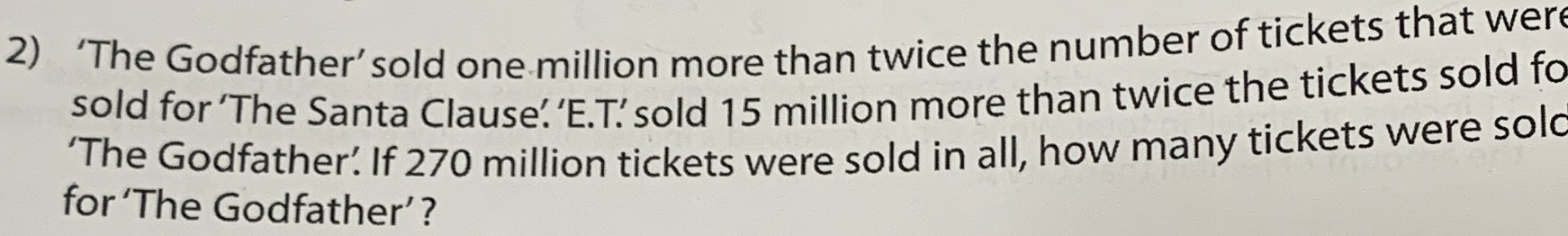### ¿Todavía tienes preguntas de matemáticas?

Pregunte a nuestros tutores expertos
Algebra
Pregunta2) 'The Godfather' sold one million more than twice the number of tickets that were sold for 'The Santa Clause'. 'E.T' sold $$15$$ million more than twice the tickets sold fo 'The Godfather'. If $$270$$ million tickets were sold in all, how many tickets were solo for 'The Godfather'?

we know nothing about the Santa Clause.

So lets take it as 'x'

Godfather $$= 2x+ 1$$

E.T. $$= 2(2x+ 1)+ 15 = 4x + 2+ 15 = 4x+ 17$$

Godfather + ET + Santa Clause = 270

$$2x+ 1 + 4x+ 17 + x = 270 \\ \\7x + 18 = 270 \\ \\ 7x = 252 \\ \\x = 36$$

Santa Clause = 36 million

Godfather = 73 million

ET  = 161 million

Solución
View full explanation on CameraMath App.